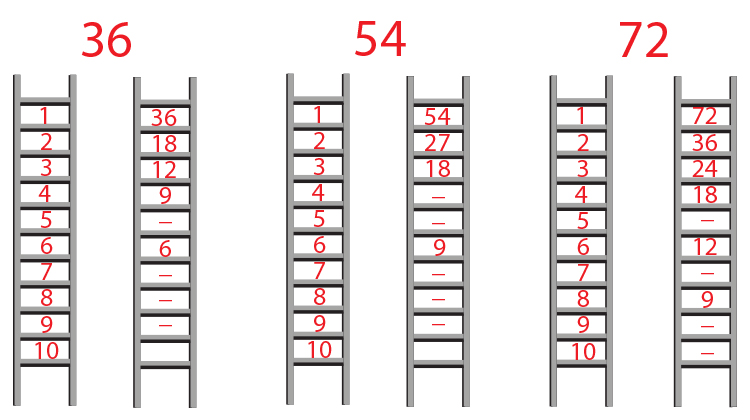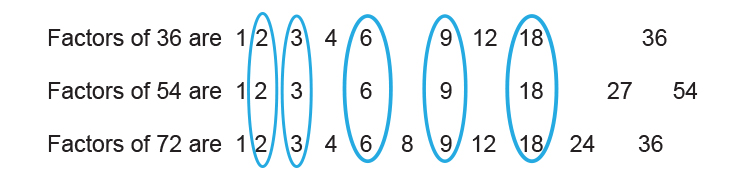# Highest common factor (HCF)

We know   Highest = Biggest number

Common = Anything the same

Factor = Take a number apart using laddersCommon ground - Is there anything the same?

So logically

Highest common factor are:Example 1

What is the highest common factor of the numbers 12 and 30?

Highest common factor = i. Take apart using ladders

ii. What have they in common

iii. Choose the biggest number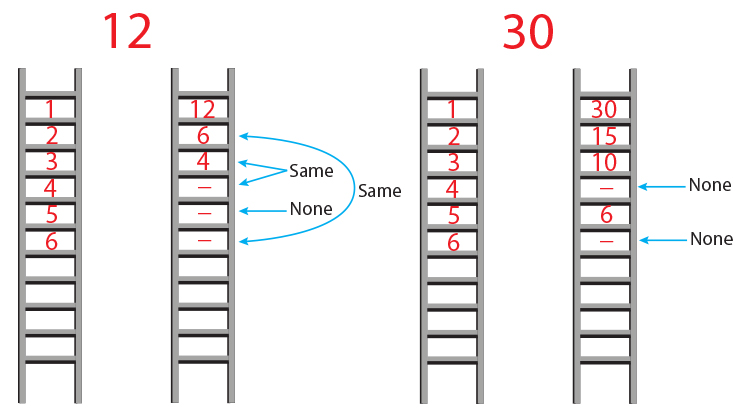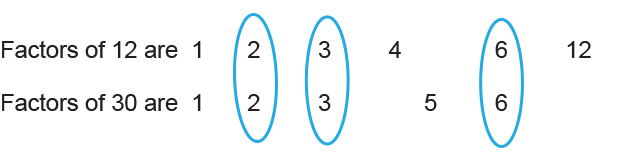ii. What they have in common
are 2, 3 and 6.

iii. The biggest number is 6.

The highest common factor of 12 and 30 is 6.

Example 2

What is the highest common factor of the numbers 12 and 15?

Highest common factor = i. Take apart using ladders

ii. What have they in common

iii. Choose the biggest number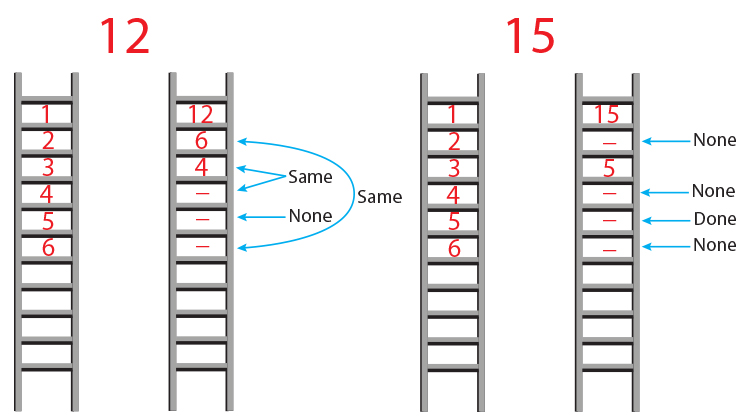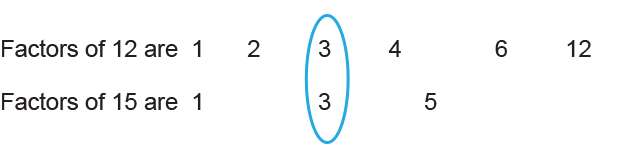ii. What they have in common
only 3.

iii. This makes 3 the biggest number too.

The highest common factor of 12 and 15 is 3.

Example 3

What is the highest common factor of the numbers 60 and 72?

Highest common factor = i. Take apart using ladders

ii. What have they in common

iii. Choose the biggest number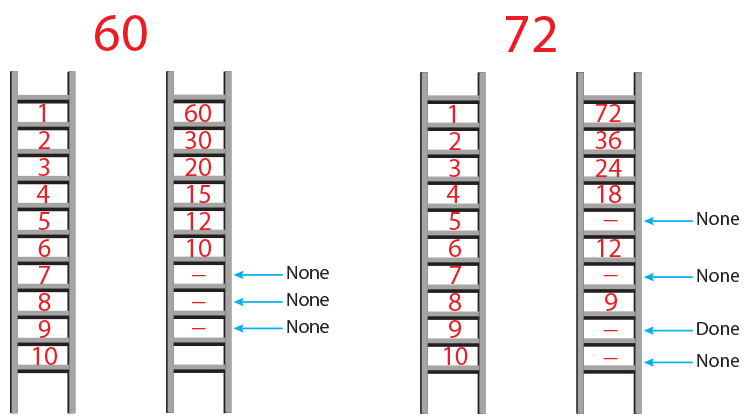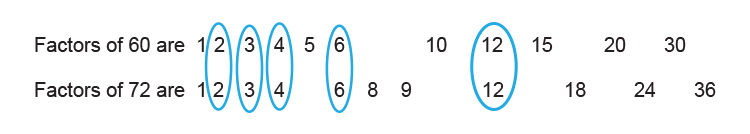ii. What they have in common is
2, 3, 6 and 12.

iii. The biggest number is 12.

The highest common factor of 60 and 72 is 12.

Example 4

What is the highest common factor of the numbers 36, 54 and 72?

Highest common factor = i. Take apart using ladders

ii. What have they in common

iii. Choose the biggest number